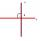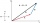Cone

If the segment of the line y = -3x +4 that lies in quadrant I is rotated about the y-axis, a cone is formed.

What is the volume of the cone?

Result

V =  7.4

Solution:Leave us a comment of example and its solution (i.e. if it is still somewhat unclear...):Be the first to comment!To solve this example are needed these knowledge from mathematics:

For Basic calculations in analytic geometry is helpful line slope calculator. From coordinates of two points in the plane it calculate slope, normal and parametric line equation(s), slope, directional angle, direction vector, the length of segment, intersections the coordinate axes etc.

Next similar examples:

1. Slope formFind the equation of a line given the point X(8, 1) and slope -2.8. Arrange your answer in the form y = ax + b, where a, b are the constants.
2. Midpoint of segmentPoint A has coordinates [-16; 23] and the midpoint of the segment AB is the point [2; 12]. What are the coordinates of point B?
3. Line segmentFor the line segment whose endpoints are L[-1, 13] and M[18, 2], find the x and y value for the point located 4 over 7 the distance from L to M.
4. LineIt is true that the lines that do not intersect are parallel?
5. V - slopeThe slope of the line whose equation is -3x -9 = 0 is
6. PerpendicularDetermine the slope of the line perpendicular to the line p: y = -x +4.
7. LineLine p passing through A[-10, 6] and has direction vector v=(3, 2). Is point B[7, 30] on the line p?
8. SlopeFind the slope of the line: x=t and y=1+t.
9. Cube cornersFrom cube of edge 14 cm cut off all vertices so that each cutting plane intersects the edges 1 cm from the nearest vertice. How many edges will have this body?
10. Two equationsSolve equations (use adding and subtracting of linear equations): -4x+11y=5 6x-11y=-5
11. Theorem proveWe want to prove the sentence: If the natural number n is divisible by six, then n is divisible by three. From what assumption we started?
12. Functions f,gFind g(1) if g(x) = 3x - x2 Find f(5) if f(x) = x + 1/2
13. TetrahedronCalculate height and volume of a regular tetrahedron whose edge has a length 18 cm.
14. PowersExpress the expression ? as the n-th power of the base 10.
15. Nineteenth memberFind the nineteenth member of the arithmetic sequence: a1=33 d=5 find a19
16. Vector - basic operationsThere are given points A [-9; -2] B [2; 16] C [16; -2] and D [12; 18] a. Determine the coordinates of the vectors u=AB v=CD s=DB b. Calculate the sum of the vectors u + v c. Calculate difference of vectors u-v d. Determine the coordinates of the vector w.
17. Coordinates of vectorDetermine the coordinate of a vector u=CD if C(19;-7) and D(-16;-5)